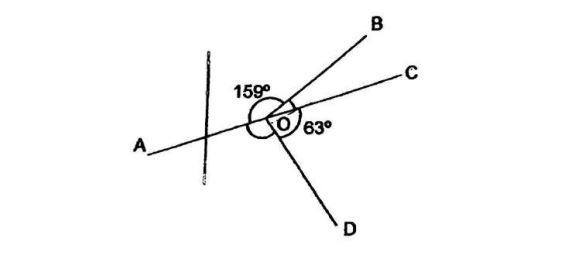# Question 1 of 100

In the figure below, AOC is a straight line $\angle$AOB = 159$^\circ$ and $\angle$COD = 63$^\circ$. What is the sum of$\angle$ AOD and$\angle$BOC?A
136$^\circ$
B
138$^\circ$
C
140$^\circ$
D
142$^\circ$
E
None of the above